# findNodes

Find mesh nodes in specified region

## Syntax

``nodes = findNodes(mesh,"region",RegionType,RegionID)``
``nodes = findNodes(mesh,"box",xlim,ylim)``
``nodes = findNodes(mesh,"box",xlim,ylim,zlim)``
``nodes = findNodes(mesh,"radius",center,radius)``
``nodes = findNodes(mesh,"nearest",point)``

## Description

example

````nodes = findNodes(mesh,"region",RegionType,RegionID)` returns the IDs of the mesh nodes that belong to the specified geometric region.```

example

````nodes = findNodes(mesh,"box",xlim,ylim)` returns the IDs of the mesh nodes within a bounding box specified by `xlim` and `ylim`. Use this syntax for 2-D meshes.```
````nodes = findNodes(mesh,"box",xlim,ylim,zlim)` returns the IDs of the mesh nodes located within a bounding box specified by `xlim`, `ylim`, and `zlim`. Use this syntax for 3-D meshes.```

example

````nodes = findNodes(mesh,"radius",center,radius)` returns the IDs of mesh nodes located within a circle (for 2-D meshes) or sphere (for 3-D meshes) specified by `center` and `radius`.```

example

````nodes = findNodes(mesh,"nearest",point)` returns the IDs of mesh nodes closest to a query point or multiple query points with Cartesian coordinates specified by `point`.```

## Examples

collapse all

Find the nodes associated with a geometric region.

Create a PDE model.

`model = createpde;`

Include the geometry of the built-in function `lshapeg`. Plot the geometry.

```geometryFromEdges(model,@lshapeg); pdegplot(model,"FaceLabels","on","EdgeLabels","on")```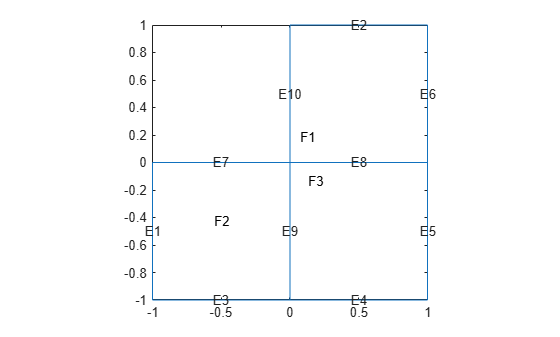Generate a mesh.

`mesh = generateMesh(model,"Hmax",0.5);`

Find the nodes associated with face 2.

`Nf2 = findNodes(mesh,"region","Face",2);`

Highlight these nodes in green on the mesh plot.

```figure pdemesh(model,"NodeLabels","on") hold on plot(mesh.Nodes(1,Nf2),mesh.Nodes(2,Nf2),"ok","MarkerFaceColor","g") ```Find the nodes associated with edges 5 and 7.

`Ne57 = findNodes(mesh,"region","Edge",[5 7]);`

Highlight these nodes in green on the mesh plot.

```figure pdemesh(model,"NodeLabels","on") hold on plot(mesh.Nodes(1,Ne57),mesh.Nodes(2,Ne57),"or","MarkerFaceColor","g")```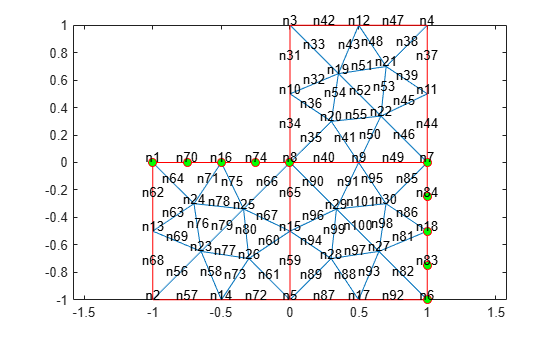Find the nodes located within a specified box.

Create a PDE model.

`model = createpde;`

Import and plot the geometry.

```importGeometry(model,"PlateHolePlanar.stl"); pdegplot(model)```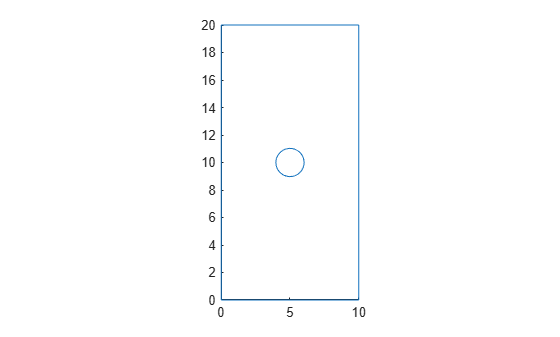Generate a mesh.

```mesh = generateMesh(model,"Hmax",2,"Hmin",0.4, ... "GeometricOrder","linear");```

Find the nodes located within the following box.

`Nb = findNodes(mesh,"box",[5 10],[10 20]);`

Highlight these nodes in green on the mesh plot.

```figure pdemesh(model) hold on plot(mesh.Nodes(1,Nb),mesh.Nodes(2,Nb),"or","MarkerFaceColor","g")```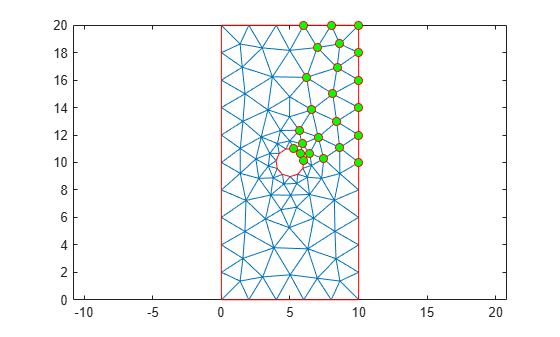Find the nodes located within a specified disk.

Create a PDE model.

`model = createpde;`

Import and plot the geometry.

```importGeometry(model,"PlateHolePlanar.stl"); pdegplot(model)```Generate a mesh.

```mesh = generateMesh(model,"Hmax",2,"Hmin",0.4, ... "GeometricOrder","linear");```

Find the nodes located within radius 2 from the center [5 10].

`Nb = findNodes(mesh,"radius",[5 10],2);`

Highlight these nodes in green on the mesh plot.

```figure pdemesh(model) hold on plot(mesh.Nodes(1,Nb),mesh.Nodes(2,Nb),"or","MarkerFaceColor","g")```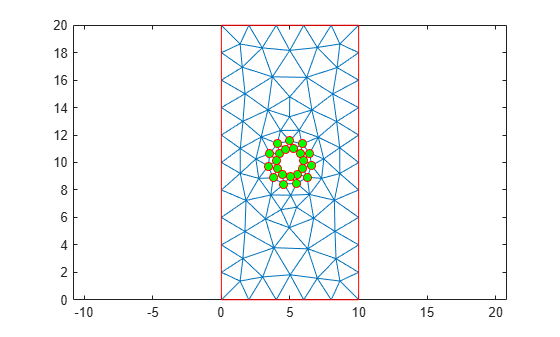Find the node closest to a specified point and highlight it on the mesh plot.

Create a PDE model.

`model = createpde;`

Import and plot the geometry.

```importGeometry(model,"PlateHolePlanar.stl"); pdegplot(model)```Generate a mesh.

`mesh = generateMesh(model,"Hmax",2,"Hmin",0.4);`

Find the node closest to the point [15;10].

`N_ID = findNodes(mesh,"nearest",[15;10])`
```N_ID = 10 ```

Highlight this node in green on the mesh plot.

```figure pdemesh(model) hold on plot(mesh.Nodes(1,N_ID),mesh.Nodes(2,N_ID),"or","MarkerFaceColor","g")```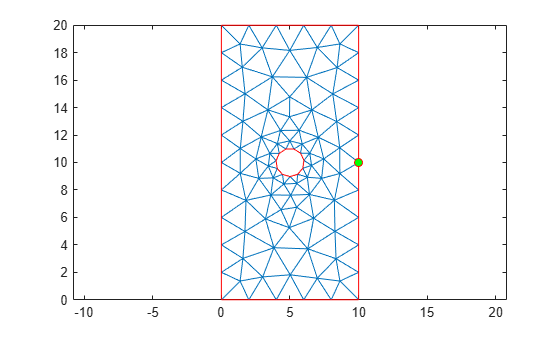## Input Arguments

collapse all

Mesh object, specified as the `Mesh` property of a `PDEModel` object or as the output of `generateMesh`.

Example: `model.Mesh`

Geometric region type, specified as `"Cell"`, `"Face"`, `"Edge"`, or `"Vertex"`.

Example: `findNodes(mesh,"region","Face",1:3)`

Data Types: `char`

Geometric region ID, specified as a vector of positive integers. Find the region IDs by using `pdegplot`.

Example: `findNodes(mesh,"region","Face",1:3)`

Data Types: `double`

x-limits of the bounding box, specified as a two-element row vector. The first element of `xlim` is the lower x-bound, and the second element is the upper x-bound.

Example: ```findNodes(mesh,"box",[5 10],[10 20])```

Data Types: `double`

y-limits of the bounding box, specified as a two-element row vector. The first element of `ylim` is the lower y-bound, and the second element is the upper y-bound.

Example: ```findNodes(mesh,"box",[5 10],[10 20])```

Data Types: `double`

z-limits of the bounding box, specified as a two-element row vector. The first element of `zlim` is the lower z-bound, and the second element is the upper z-bound. You can specify `zlim` only for 3-D meshes.

Example: ```findNodes(mesh,"box",[5 10],[10 20],[1 2])```

Data Types: `double`

Center of the bounding circle or sphere, specified as a two-element row vector for a 2-D mesh or three-element row vector for a 3-D mesh. The elements of these vectors contain the coordinates of the center of a circle or a sphere.

Example: ```findNodes(mesh,"radius",[0 0 0],0.5)```

Data Types: `double`

Radius of the bounding circle or sphere, specified as a positive number.

Example: ```findNodes(mesh,"radius",[0 0 0],0.5)```

Data Types: `double`

Cartesian coordinates of query points, specified as a 2-by-N or 3-by-N matrix. These matrices contain the coordinates of the query points. Here, N is the number of query points.

Example: ```findNodes(mesh,"nearest",[15 10.5 1; 12 10 1.2])```

Data Types: `double`

## Output Arguments

collapse all

Node IDs, returned as a positive integer or a row vector of positive integers.

## Version History

Introduced in R2018a